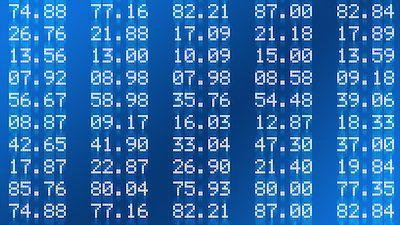The Most Common Rounding Methods

When you are looking to round numbers, then you need to know that you can use different methods. The truth is that you have multiple rounding methods and choosing the best one depends on your goals.

Learn to round all the numbers you need.

One of the things to keep in mind is that most of the time, you are said that when you want to round a number, you should do it to the nearest. And this is the most popular rounding method that there is. But today, we also want to ensure that you get to know other rounding methods that you can use.

#1: Round To The Nearest:

As we just mentioned above, this is the most popular and most commonly used rounding method.

Overall, you just need to know that when you have a number that is less than 5, you will need to round the number down. On the other hand, when you have a number that is equal to 5 or greater, then you will need to round the number up.

Rounding numbers to the nearest hundred.

#2: Round Half Up And Down:

This is also a very popular rounding method that you can use when you want or need to round numbers.

The truth, and generally speaking, you just need to know that you will be rounding values that are halfway between the chosen rounding precision up. For example, when you want to round up the number 5.50 to ones place, then you would get 6. On the other hand, if you wanted yo round down the same number to ones place, you would get 5.

So, what about the number 5.51? In this case, if you wanted to round it up to ones place, you would get 6 and if you wanted to round it down to ones place, you would also get 6.

Discover how to round numbers to the nearest hundredth.

#3: Round Up (Ceiling) And Round Down (Floor):

Up until now, we have mentioned two of the most popular rounding methods. And while the round up (ceiling) and round down (floor) isn’t that popular, it can still be very handy.

The only thing that you should keep in mind when you are using this rounding method is the fact that you will either be rounding the number up or down towards the nearest integer.

So, let’s check an example. Let’s say that you want to round the number 8.01 using this rounding method. In case you want to round up (ceiling), you will get 9. On the other hand, in case you want to round down (floor), you will get 8. As you can see, this rounding method is also very easy to use.

Don’t know how to round to nearest tens?

#4: Round Half To Odd Or Round Half To Even:

These rounding methods tend to be used mainly as tie-breaking rules. Notice that when you are using these rounding methods, you won’t need to worry about positive or negative numbers as well as you won’t also need to worry about rounding away or towards zero. In fact, you will only need to round the numbers to the nearest odd or even integer depending on what you want.

Let’s say that you want to round the number 6.5 using these rounding methods. In case you want to round half to odd, then you will get 7. On the other hand, if you want to round half to even, then you will get 6.